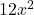## Which expression is equal to 12x A. 4x + 4x – 4x B. 24x/2 C. (3x)(4x) D. 15x – 3​

Question

Which expression is equal to 12x
A. 4x + 4x – 4x
B. 24x/2
C. (3x)(4x)
D. 15x – 3​

in progress 0
6 months 2021-09-04T11:53:46+00:00 2 Answers 0 views 0

B.

Step-by-step explanation:

4x + 4x – 4x = 4x

24x ÷ 2 = 12x

3x • 4x =15x – 3 = 15x – 3

B. 24x/2

Step-by-step explanation:

12x

A. 4x + 4x – 4x = 8x – 4x = 4x

B. 24x/2  = 24/2 x = 12x

C. (3x)(4x) = 12 x^2

D. 15x – 3​ = 15x -3  ( no like terms